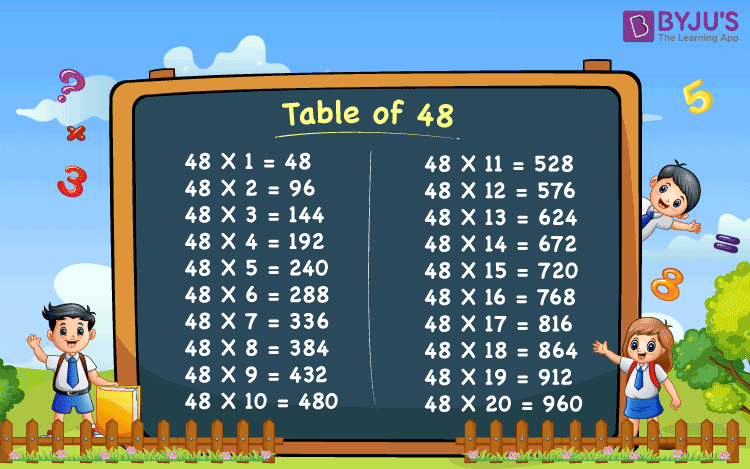# Table of 48

Table of 48 represents the repeated addition of number 48 when we multiply it to another number. For example, 48 multiplied by 3 can be expressed as:

48 x 3 ⇒ 48+ 48+ 48= 144

In the same way, we can find the other multiples of 48 by repeatedly adding them. But this method is long. So, it is always better to learn and memorise the tables for ease of calculations. Learn the tables online for free and solve arithmetic problems in a quick way at BYJU’S. Also, check Tables from 1 to 100 for more details.

## Table of 48 Chart## Multiplication Table of 48

Maths multiplication tables are the fundamentals, which we learn since our primary classes. Memorise the Table of 48 given below up to 20 times, in a tabular form. Also, these tables are very useful for exam preparation and revisions.

 48 x 1 = 48 48 x 2 = 96 48 x 3 = 144 48 x 4 = 192 48 x 5 = 240 48 x 6 = 288 48 x 7 = 336 48 x 8 = 384 48 x 9 = 432 48 x 10 = 480 48 x 11 = 528 48 x 12 = 576 48 x 13 = 624 48 x 14 = 672 48 x 15 = 720 48 x 16 = 768 48 x 17 = 816 48 x 18 = 864 48 x 19 = 912 48 x 20 = 960

Let us see how we can read the table for the number 48.

## 48 Times Table

To memorise the table of forty-eight, we have presented here the format to read the table.

• One time forty-eight is 48
• Two times forty-eight is 96
• Three times forty-eight is 144
• Four times forty-eight is 192
• Five times forty-eight is 240
• Six times forty-eight is 288
• Seven times forty-eight is 336
• Eight times forty-eight is 384
• Nine times forty-eight is 432
• Ten times forty-eight is 480

Practise writing the Table of 48, in the above format from 11 to 20 times and become an expert.

## Solved Examples

Q.1: Find the value of 48 x 5 + 10.

Solution: 48 x 5 + 10

= (48 x 5) + 10 [BODMAS rule]

= 240 + 10

= 250

Q.2: Find the value of 48 x 11 ÷ 11.

Solution: Given, 48 x 11 ÷ 11

= 48 x (11 ÷ 11) [BODMAS rule]

= 48 x 1

= 48

Q.3: Which of these is a multiple of 48?

191, 245, 288, 338, 388, 435, 490

Solution: To check if the given numbers are the multiples of 48, we have to divide each of these numbers by 48. If the quotient after division is a whole number then it is a multiple of 48 but if it is a fraction, then it is not. Let us proceed.

191 ÷ 48= 3.97.. (fraction)

245 ÷ 48= 5.10.. (fraction)

288 ÷ 48= 6 (whole number)

338 ÷ 48= 7.04.. (fraction)

388 ÷ 48= 8.08.. (whole number)

435 ÷ 48= 9.06.. (fraction)

490 ÷ 48= 10.2.. (fraction)

Therefore, we can see, only 288 is completely divisible by 48, giving the value of quotient as 6. Thus, it is the required multiple.

### Check More Tables

For more knowledge on Multiplication Table subscribe to BYJU’S. Also, download BYJU’S-The Learning App for conceptual and interactive videos.

## Frequently Asked Questions on Table of 48

### What is Table of 48?

Table of 48 represents the values that are repeated addition of 48.
48 x 1 = 48
48 x 2 = 48+ 48= 96
48 x 3 = 48+ 48+ 48= 144
48 x 4 = 48+ 48+ 48+ 48= 192
And so on.

### What are the multiples of 48?

The first ten multiples of 48 are 48, 96, 144, 192, 240, 288, 336, 384, 432 and 480.

### What is the sum of the first five multiples of 48?

The sum of the first five multiples of 48 is 720.

### What is the average of the first three multiples of 48?

The first three multiples are 48, 96 and 144.
Average = (Sum of all multiples) ÷ Number of multiples
= (48+ 96+ 144) ÷ 3
= 288 ÷ 3
= 96

### How many factors does the number 48 have?

The factors of 48 are 1, 2, 3, 4, 6, 8, 12, 16, 24 and 48. Thus, there are 10 factors for the number 48.